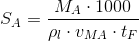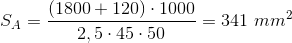## Gate area

Cross section of the opening the casting metal flows through with vertical position to the flow direction. For rectangular gate areas, the gate area is the product from gate length and gate thickness. Example: Approximate calculation of the gate area for die cast parts according to Eq. 1:

Eq. 1:sA = Gate area in mm²
mA = Weight of the casting from the gate (incl. overflows and vacuum channel) in g
ρl = Density of the metal in liquid phase at casting temperature in g/cm³
vMA = Gate speed in m/s
tF = Mold filling time in ms

For a casting with a parts weight of 1,800 g and a overflow weight of 120 g made of the alloy Al Si9Cu3(Fe) (ρl=2.5 g/cm³) and a defined gate speed of 45 m/s and a required mold filling time of 50 ms, Eq. 2 defines a total gate area of:

Eq. 2:From a total number of 6 potential gates at the casting and a casting thickness of 1.2 mm recommended for the alloy, six rectangular individual cross sections with the dimensions 1.2 x 48 mm are derived. For gray cast iron, W Schreitel has prepared a diagram to determine the gate areas (see Schreitel diagram).